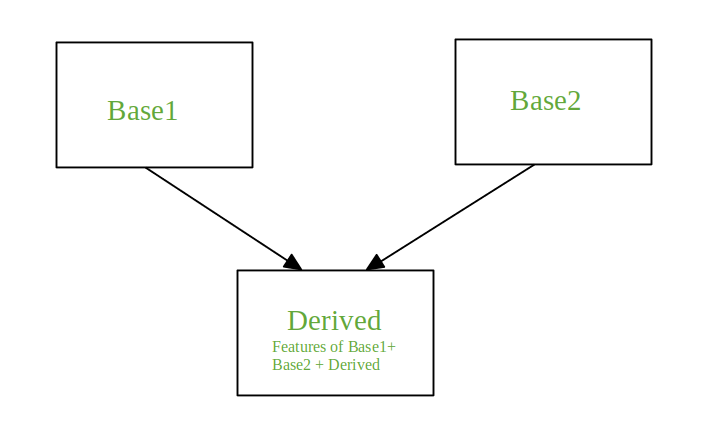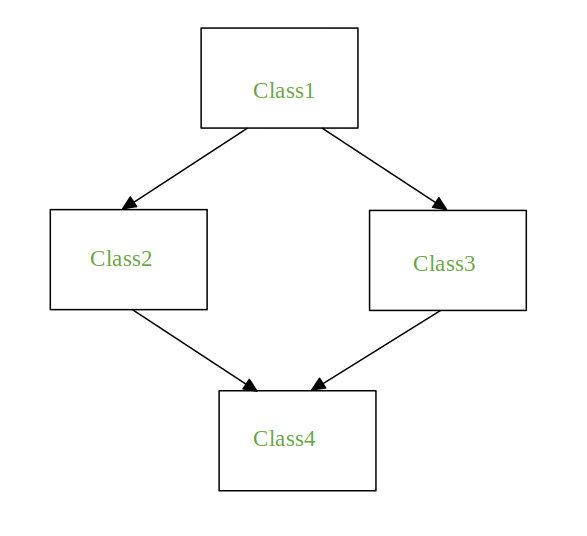# Multiple Inheritance in Python

## Multiple Inheritance in Python

Inheritance is the mechanism to achieve the re-usability of code as one class(child class) can derive the properties of another class(parent class). It also provides transitivity ie. if class C inherits from P then all the sub-classes of C would also inherit from P.
Multiple Inheritance
When a class is derived from more than one base class it is called multiple Inheritance. The derived class inherits all the features of the base case.```Syntax:

Class Base1:
Body of the class

Class Base2:
Body of the class

Class Derived(Base1, Base2):
Body of the class```

In the coming section, we will see the problem faced during multiple inheritance and how to tackle it with the help of examples.
The Diamond ProblemIt refers to an ambiguity that arises when two classes Class2 and Class3 inherit from a superclass Class1 and class Class4 inherits from both Class2 and Class3. If there is a method “m” which is an overridden method in one of Class2 and Class3 or both then the ambiguity arises which of the method “m” Class4 should inherit.
When the method is overridden in both classes

 `# Python Program to depict multiple inheritance` `# when method is overridden in both classes` `class` `Class1:` `    ``def` `m(``self``):` `        ``print``(``"In Class1"``)` `      ` `class` `Class2(Class1):` `    ``def` `m(``self``):` `        ``print``(``"In Class2"``)` `class` `Class3(Class1):` `    ``def` `m(``self``):` `        ``print``(``"In Class3"``) ` `       ` `class` `Class4(Class2, Class3):` `    ``pass` `    ` `obj ``=` `Class4()` `obj.m()`

Output:

`In Class2`

Note: When you call obj.m() (m on the instance of Class4) the output is In Class2. If Class4 is declared as Class4(Class3, Class2) then the output of obj.m() will be In Class3.
When the method is overridden in one of the classes

 `# Python Program to depict multiple inheritance` `# when method is overridden in one of the classes` `class` `Class1:` `    ``def` `m(``self``):` `        ``print``(``"In Class1"``)` `      ` `class` `Class2(Class1):` `    ``pass` `class` `Class3(Class1):` `    ``def` `m(``self``):` `        ``print``(``"In Class3"``)   ` `     ` `class` `Class4(Class2, Class3):` `    ``pass` `obj ``=` `Class4()` `obj.m()`

Output:

`In Class3`

When every class defines the same method

 `# Python Program to depict multiple inheritance` `# when every class defines the same method` `class` `Class1:` `    ``def` `m(``self``):` `        ``print``(``"In Class1"``)` `      ` `class` `Class2(Class1):` `    ``def` `m(``self``):` `        ``print``(``"In Class2"``)` `class` `Class3(Class1):` `    ``def` `m(``self``):` `         ``print``(``"In Class3"``)    ` `    ` `class` `Class4(Class2, Class3):` `    ``def` `m(``self``):` `        ``print``(``"In Class4"``)  ` `obj ``=` `Class4()` `obj.m()` `Class2.m(obj)` `Class3.m(obj)` `Class1.m(obj)`

Output:

```In Class4
In Class2
In Class3
In Class1```

The output of the method obj.m() in the above code is In Class4. The method “m” of Class4 is executed. To execute the method “m” of the other classes it can be done using the class names.
Now, to call the method m for Class1, Class2, Class3 directly from the method “m” of the Class4 see the below example

 `# Python Program to depict multiple inheritance` `# when we try to call the method m for Class1,` `# Class2, Class3 from the method m of Class4` ` ` `class` `Class1:` `    ``def` `m(``self``):` `        ``print``(``"In Class1"``) ` `     ` `class` `Class2(Class1):` `    ``def` `m(``self``):` `        ``print``(``"In Class2"``)` `class` `Class3(Class1):` `    ``def` `m(``self``):` `        ``print``(``"In Class3"``)    ` `    ` `class` `Class4(Class2, Class3):` `    ``def` `m(``self``):` `        ``print``(``"In Class4"``)  ` `        ``Class2.m(``self``)` `        ``Class3.m(``self``)` `        ``Class1.m(``self``)` `obj ``=` `Class4()` `obj.m()`

Output:

```In Class4
In Class2
In Class3
In Class1```

To call “m” of Class1 from both “m” of Class2 and “m” of Class3 instead of Class4 is shown below:

 `# Python Program to depict multiple inheritance` `# when we try to call m of Class1 from both m of` `# Class2 and m of Class3` `class` `Class1:` `    ``def` `m(``self``):` `        ``print``(``"In Class1"``)  ` `    ` `class` `Class2(Class1):` `    ``def` `m(``self``):` `        ``print``(``"In Class2"``)` `        ``Class1.m(``self``)` `class` `Class3(Class1):` `    ``def` `m(``self``):` `        ``print``(``"In Class3"``)` `        ``Class1.m(``self``)  ` `     ` `class` `Class4(Class2, Class3):` `    ``def` `m(``self``):` `        ``print``(``"In Class4"``)  ` `        ``Class2.m(``self``)` `        ``Class3.m(``self``)` `     ` `obj ``=` `Class4()` `obj.m()`

Output:

```In Class4
In Class2
In Class1
In Class3
In Class1```

The output of the above code has one problem associated with it, the method m of Class1 is called twice. Python provides a solution to the above problem with the help of the super() function. Let’s see how it works.
The super Function

 `# Python program to demonstrate` `# super()` `class` `Class1:` `    ``def` `m(``self``):` `        ``print``(``"In Class1"``)` `class` `Class2(Class1):` `    ``def` `m(``self``):` `        ``print``(``"In Class2"``)` `        ``super``().m()` `class` `Class3(Class1):` `    ``def` `m(``self``):` `        ``print``(``"In Class3"``)` `        ``super``().m()` `class` `Class4(Class2, Class3):` `    ``def` `m(``self``):` `        ``print``(``"In Class4"``)  ` `        ``super``().m()` `     ` `obj ``=` `Class4()` `obj.m()`

Output:

```In Class4
In Class2
In Class3
In Class1```

Super() is generally used with the __init__ function when the instances are initialized. The super function comes to a conclusion, on which method to call with the help of the method resolution order (MRO).

### Method resolution order:

In Python, every class whether built-in or user-defined is derived from the object class and all the objects are instances of the class object. Hence, the object class is the base class for all the other classes.
In the case of multiple inheritance, a given attribute is first searched in the current class if it’s not found then it’s searched in the parent classes. The parent classes are searched in a depth-first, left-right fashion and each class is searched once.
If we see the above example then the order of search for the attributes will be Derived, Base1, Base2, object. The order that is followed is known as a linearization of the class Derived and this order is found out using a set of rules called Method Resolution Order (MRO).
To view the MRO of a class:

• Use the mro() method, it returns a list
Eg. Class4.mro()
• Use the _mro_ attribute, it returns a tuple
Eg. Class4.__mro__

Example:

 `# Python program to demonstrate` `# super()` `class` `Class1:` `    ``def` `m(``self``):` `        ``print``(``"In Class1"``)` `class` `Class2(Class1):` `    ``def` `m(``self``):` `        ``print``(``"In Class2"``)` `        ``super``().m()` `class` `Class3(Class1):` `    ``def` `m(``self``):` `        ``print``(``"In Class3"``)` `        ``super``().m()` `class` `Class4(Class2, Class3):` `    ``def` `m(``self``):` `        ``print``(``"In Class4"``)  ` `        ``super``().m()` `     ` `print``(Class4.mro())         ``#This will print list` `print``(class4.__mro__)        ``#This will print tuple`

Output:

[<class ‘__main__.Class4’>, <class ‘__main__.Class2’>, <class ‘__main__.Class3’>, <class ‘__main__.Class1’>, <class ‘object’>]
(<class ‘__main__.Class4’>, <class ‘__main__.Class2’>, <class ‘__main__.Class3’>, <class ‘__main__.Class1’>, <class ‘object’>)

Last Updated on November 13, 2021 by admin

## Removing stop words with NLTK in PythonRemoving stop words with NLTK in Python

Removing stop words with NLTK in Python The process of converting data to something a

## Append to JSON file using PythonAppend to JSON file using Python

Append to JSON file using Python The full form of JSON is JavaScript Object Notation.

## Python – Initialize empty array of given lengthPython – Initialize empty array of given length

Python – Initialize empty array of given length As we know Array is a collection of items

## How to convert timestamp string to datetime object in Python?How to convert timestamp string to datetime object in Python?

How to convert timestamp string to datetime object in Python? Python has a module named DateTime to

## Python OpenCV – cv2.rectangle() methodPython OpenCV – cv2.rectangle() method

Python OpenCV | cv2.rectangle() method OpenCV-Python is a library of Python bindings designed to solve computer

## string.punctuation in Pythonstring.punctuation in Python

string.punctuation in Python In Python3, string.punctuation is a pre-initialized string used as string constant. In Python, string.punctuation will give

## range() vs xrange() in Pythonrange() vs xrange() in Python

range() vs xrange() in Python The range() and xrange() are two functions that could be

## Types of inheritance PythonTypes of inheritance Python

Types of inheritance Python Inheritance is defined as the capability of one class to derive